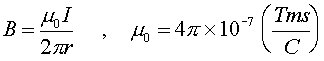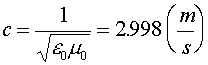Magnetic field of a long wire Magnetic fields arise from charges, similarly to electric fields, but are different in that the charges must be moving. A long straight wire carrying a current is the simplest example of a moving charge that generates a magnetic field. We mentioned that the force a charge felt when moving through a magnetic field depended on the right-hand rule. The direction of the magnetic field due to moving charges will also depend on the right hand rule. For the case of a long straight wire carrying a current I, the magnetic field lines wrap around the wire. By pointing one's right thumb along the direction of the current, the direction of the magnetic field can by found by curving one's fingers around the wire.The strength of the magnetic field depends on the current I in the wire and r, the distance from the wire.The constant m0 is the magnetic permiability. The reason is does not appear as an arbitray number is that the units of charge and current (coulombs and amps) were chosen to give a simple form for this constant. One can also notice the the product of m0 and e0 are related to the velocity of light. (More on that later, fundamental constants)If one remembers the case of the electric field of a uniformly charged wire, it also fell as 1/r. There is no real analogy to coulombs law for magnetism, as the magnetic field of a point charge is complicated since it can't be standing still to generate a magnetic field.